# ML Aggarwal Class 10 Solutions for ICSE Maths Chapter 21 Measures of Central Tendency Ex 21.2

## ML Aggarwal Class 10 Solutions for ICSE Maths Chapter 21 Measures of Central Tendency Ex 21.2

ML Aggarwal Class 10 Solutions for ICSE Maths Chapter 21 Measures of Central Tendency Ex 21.2

Question 1.
A student scored the following marks in 11 questions of a question paper: 3, 4, 7, 2, 5, 6, 1, 8, 2, 5, 7 Find the median marks.
Solution: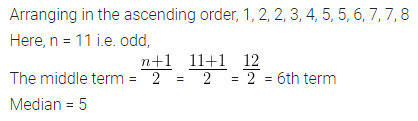Question 2.
(a) Find the median of the following set of numbers: 9, 0, 2, 8, 5, 3, 5, 4, 1, 5, 2, 7 (1990)
(b) For the following set of numbers, find the median: 10, 75, 3, 81, 17, 27, 4, 48, 12, 47, 9, 15.
Solution: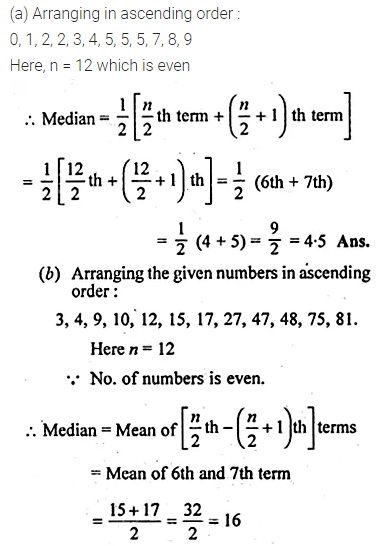Question 3.
Calculate the mean and the median of the numbers: 2, 1, 0, 3, 1, 2, 3, 4, 3, 5
Solution: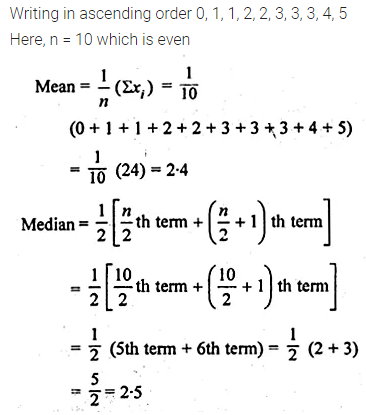Question 4.
The median of the observations 11, 12, 14, (x – 2), (x + 4), (x + 9), 32, 38, 47 arranged in ascending order is 24. Find the value of x and hence find the mean.
Solution: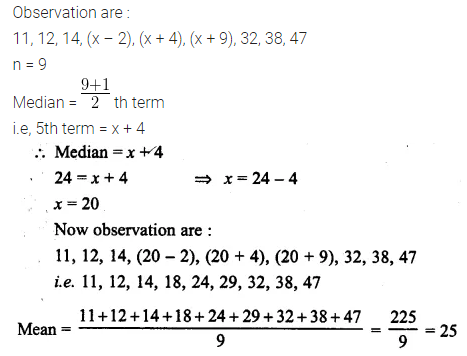Question 5.
The mean of the numbers 1, 7, 5, 3, 4, 4, is m. The numbers 3, 2, 4, 2, 3, 3, p have mean m – 1 and median q. Find
(i) p
(ii) q
(iii) the mean of p and q.
Solution: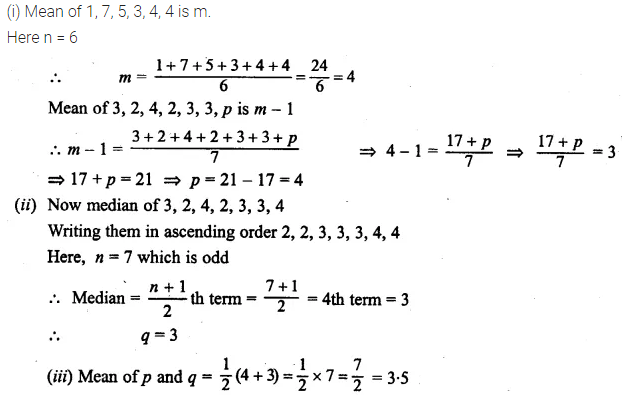Question 6.
Find the median for the following distribution: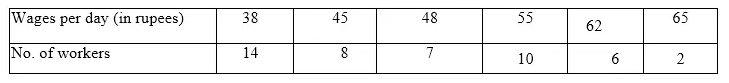Solution:
Writing the distribution in the cumulative frequency table: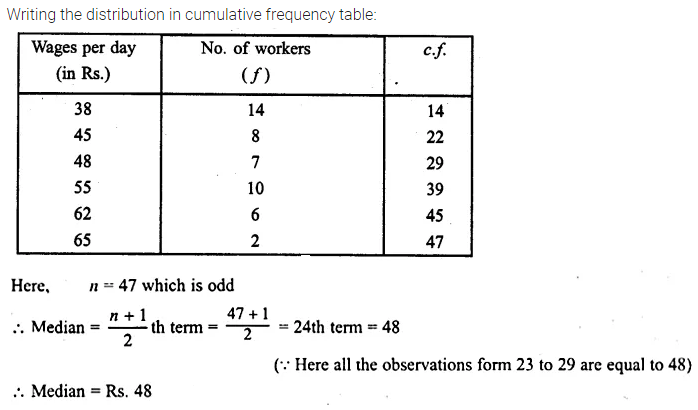Question 7.
Find the median for the following distribution.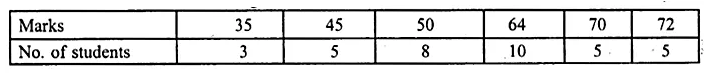Solution: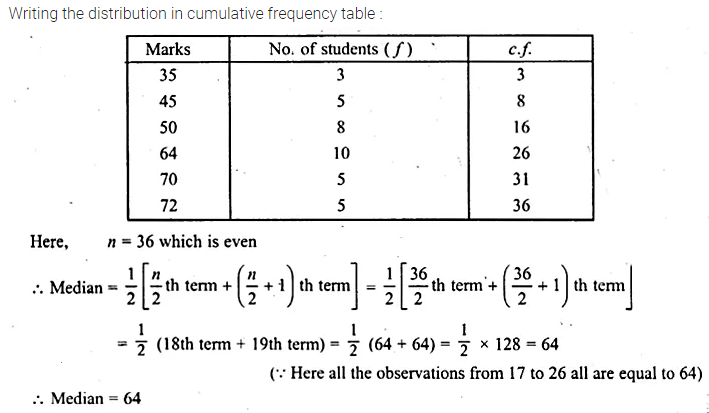Question 8.
Marks obtained by 70 students are given below:Calculate the median marks.
Solution: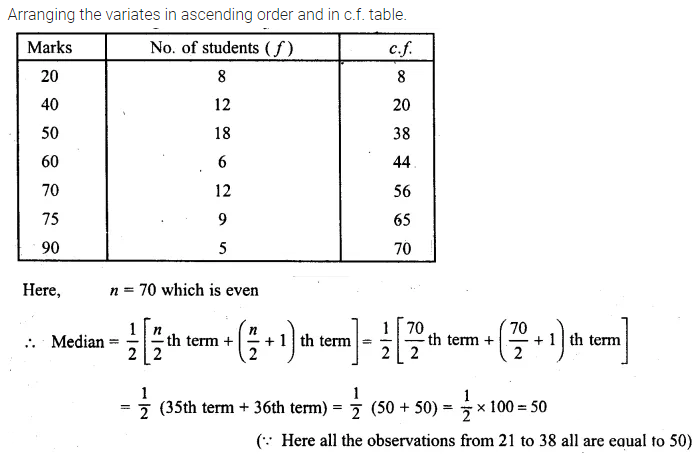Question 9.
Calculate the mean and the median for the following distribution:Solution:Question 10.
The daily wages (in rupees) of 19 workers are
41, 21, 38, 27, 31, 45, 23, 26, 29, 30, 28, 25, 35, 42, 47, 53, 29, 31, 35.
Find
(i) the median
(ii) lower quartile
(iii) upper quartile range,
(iv) interquartile range.
Solution:Question 11.
From the following frequency distribution, find:
(i) the median
(ii) lower quartile
(iii) upper quartile
(iv) interquartile range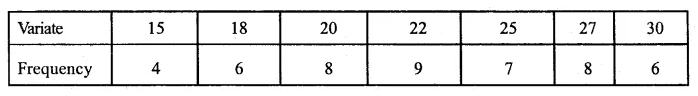Solution:Question 12.
For the following frequency distribution, find:
(i) the median
(ii) lower quartile
(iii) upper quartile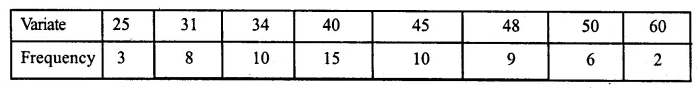Solution: